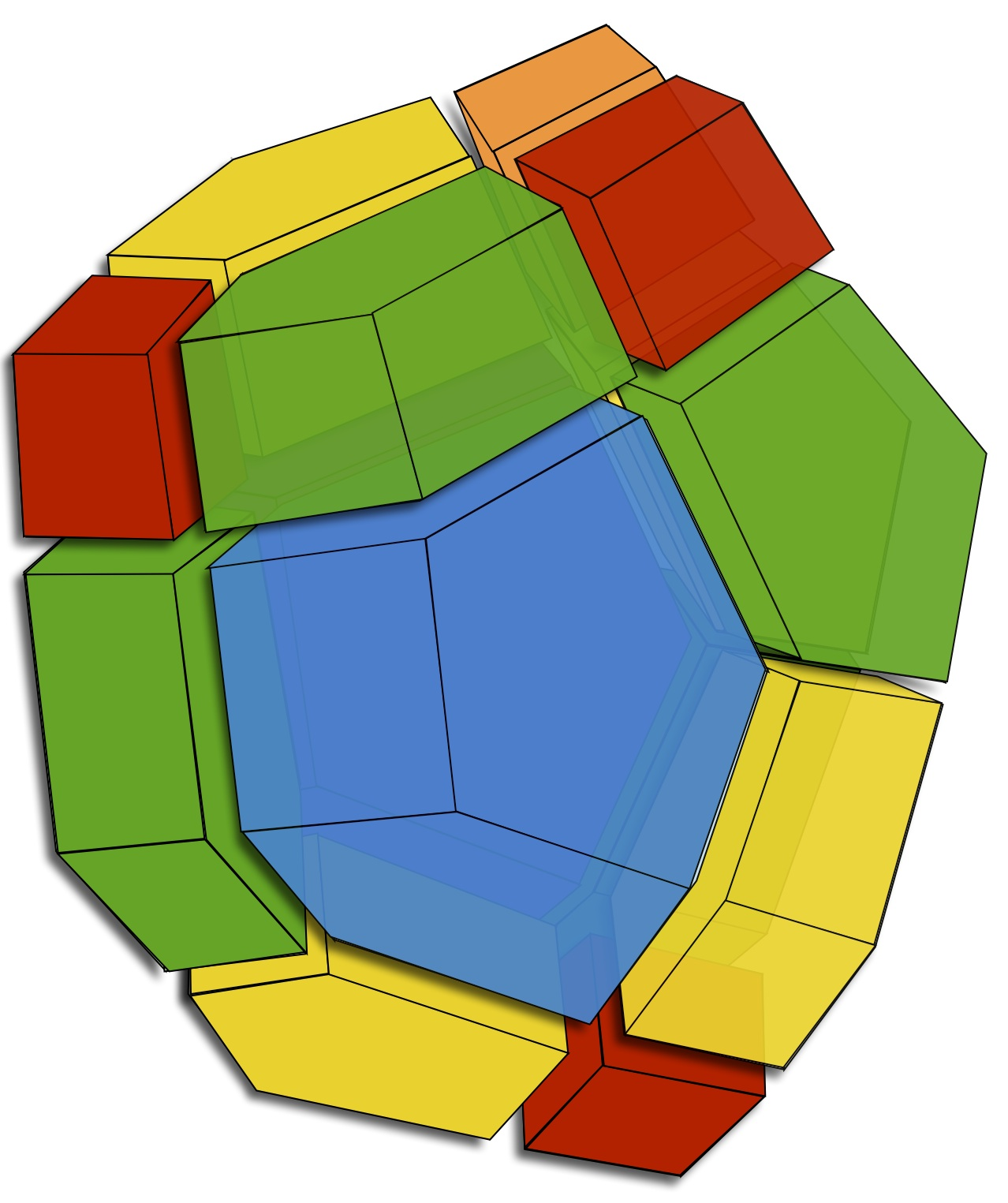# Research

My research involves the study of interesting interactions between algebraic structures (spaces of diagonal harmonic polynomials, representations of reflection groups, etc.) and combinatorial objects (trees, integer partitions, permutations, Catalan structures, parking functions, etc.). These interactions give rise to several identities, often expressed in terms of generating functions or symmetric functions (Schur functions, Macdonald polynomials, etc.). In the middle of all this lies the study of the structure of the diagonal coinvariant $\mathbb{S}_n$-module  (in two or more sets of $n$-variables). In particular, the case of three sets of variables is tied with the study of decorated intervals of a $m$-variant of the Tamari lattice (associahedron).Interval decomposition of the 2-Tamari lattice with $n=4$

All this lies at the intersection of Combinatorics, Representation Theory, and Algebraic Geometry; with natural ties to Theoretical Physics and Knot/Links Theory. In general, I make extensive use of computer algebra in my research, to explore properties of intricate algebraic structures.

I am currently exploring intriguing structural properties of $GL_\infty\times S_n$-modules of diagonal harmonic polynomials. I have recently proposed several conjectures, some regarding $q$ and $(q,t)$ generalizations of Foulkes’ conjecture, others regarding ties between Rational Catalan Combinatorics and the Elliptic Hall Algebra, and still others linked to these subjects and the study of the homology of $(m,n)$-torus knot.

Previously, I have been collaborating with Adriano Garsia, Mark Haiman and others on algebraic and combinatorial aspects of the study of $(k,n)$-parking functions. In this general context, we have a generalized shuffle conjecture. This is a continuation (with a lot of new twists) of our long-standing study of combinatorial aspects of Diagonal Harmonic Polynomials of the Symmetric Group. Macdonald eigenoperators (i.e.: those that have Macdonald polynomials as joint eigenfunctions) are nice tools for the study of many identities involving Macdonald polynomials. Among these operators, the $\nabla$ operator (that I introduced in 1994 and then studied with Adriano Garsia, Mark Haiman, and others) plays a particularly important role.

In collaboration with Nantel Bergeron, Adriano Garsia, and Christophe Reutenauer we started in the 90’s an extensive study of the structure of Solomon’s Descent Algebras of Finite Coxeter Groups. There are a lot of nice applications of the results obtained, but one of these is fun and easy to talk about. It relates to the study of cards shuffling, as discussed in this paper (see references therein) by Dave Bayer and Persi Diaconis which has received wide publicity even in the general media.

In collaboration with other members of Lacim (mainly Pierre Leroux and Gilbert Labelle), I contributed in the 80-90 to the original development of the Theory of Species (introduced by André Joyal). For an introduction to the theory, see Combinatorial Species. My collaboration with Simon Plouffe has contributed to the emergence of tools such as GFUN (in Maple).

For other aspects of my past research, look up MathSciNet or my Google Scholar Citations, or my publication page. I have also written short more technical summaries about some aspects of my research.